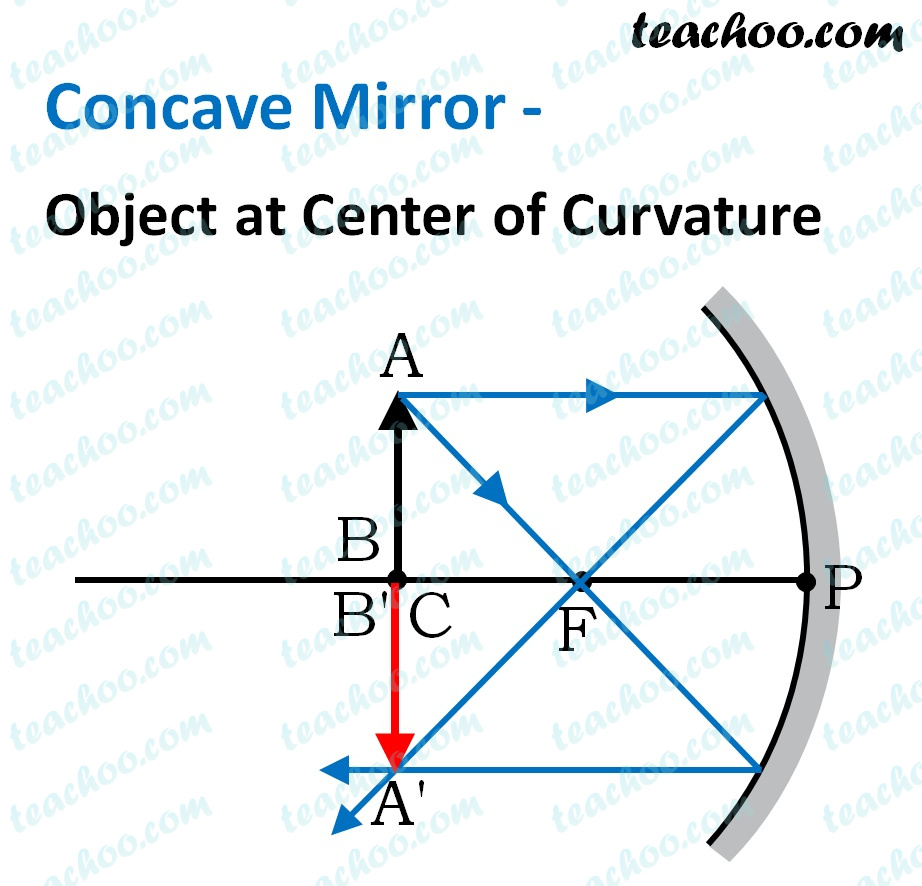Solutions - CBSE Class 10 Sample Paper for 2022 Boards - Science [MCQ]

Class 10
Solutions to CBSE Sample Paper - Science Class 10

## (D) More than 30 cm in front of the mirror

Since rays converge at a point 15 cm from the mirror, then, focal length is 15 cm .

Also, it is concave mirror, focal length will be negative.

Thus,

Focal length = f = -15 cm

Radius of Curvature = C = -30cm

For a Concave mirror

• An object kept at C makes an image of the same size as object
• Thus, the object should be kept at CSo, the correct answer is (a)

Get live Maths 1-on-1 Classs - Class 6 to 12